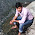## Thursday, October 11, 2012

### How to find the total count of a word / string in a file?

How to find/calculate the total count of occurences of a particular word in a file?

Let us consider a file with the following contents. The requirement is to find the total number of occurrences of the word 'Unix':
```\$ cat file
Unix is an OS
Linux is also Unix
Welcome to Unix, and Unix OS.
```
1. Using the grep command:
```\$ grep -o 'Unix' file | wc -l
4
```
The '-o' option of grep is very powerful one. As seen earlier, '-o' options prints only the part of the string which matchced the search pattern, unlike the default way of printing the entire line on matching a pattern. The output of grep will be a series of lines with the word 'Unix' and by counting the number of lines(wc -l), the total word count of 'Unix' can be obtained.
The above solution will match 'Unix', 'Unixe', 'Unixx', etc. In case, if the requirement is to match for the exact word:
```\$ grep -o '\bUnix\b' file | wc -l
4
```
The \b indicates word boundary.

2. tr command:
```\$ tr -s " " "\n" < file | grep -c Unix
4
```
Using the tr, the whole file is split into words. And hence, by doing the word count of "Unix" using the -c option of grep, the desired result is achieved.

3. awk solution:
```\$ awk '/Unix/{x++}END{print x}' RS=" " file
4
```
The key in the awk solution is the special variable RS(Record separator). By default, awk reads a line on the basis of newline character which is the record separator. By setting the RS with space, the record separator is set to whitespace which means every word is treated as a record by awk. Hence, just by incrementing a variable on encountering the pattern 'Unix', the total word count is found out.

4. Perl solution:
```\$ perl -ne '\$x+=s/Unix//g;END{print "\$x\n"}' file
4
```
Perl solution is pretty handy. The substitution command(s) of Perl returns the number of substitutions made. By substituting the pattern 'Unix' with something in every line, the total count of 'Unix' in the particular line is obtained. By accumulating this count, the total word count of 'Unix' is retrieved.

5. Another Perl solution:
```\$ perl -ane '\$x+=grep(/Unix/,@F);END{print "\$x\n";}' file
4
```
This uses the grep function of Perl. By using the auto mode(a), the entire line is read into the special array @F. By grepping for the pattern "Unix' in the array, the elements matching the pattern is retrieved. Since the result is collected in scalar, we get the count of the pattern, and hence the result.#### 1 comment:

1.grep -ic "Arun" file_name